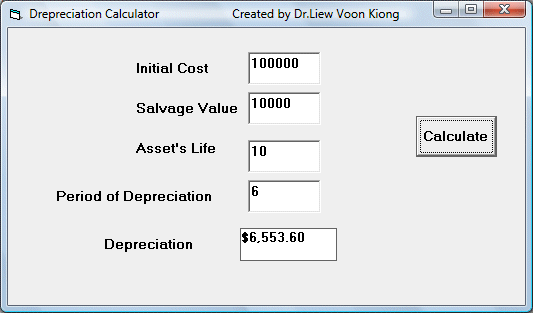# Depreciation Calculator

Depreciation means a reduction in the value of an asset with the passage of time . It is computed based on the initial purchase price or initial cost, number of years  where depreciation is calculated, salvage value at the end of the depreciation period, and the asset's life span.

Depreciation is an important element in the management of a company's assets. With proper and accurate calculation of depreciation, a company can benefit from the tax advantage.

In Visual Basic, The of the depreciation function is

DDB(Cost,Salvage,Life, Period)

Cost=Initial cost

Salvage=Salvage value

Life=Asset's life

Period=Depreciation period

In this program, we need to insert four text boxes for the user to enter the initial cost, the salvage value, the asset's life and the period of depreciation. Besides that, insert a label to display the amount of depreciation and a command button to compute the depreciation.

### The Interface### The Code

Private Sub Command1_Click()

Dim Int_Cost, Sal_Value, Asset_Life, Deperiod, Depre_Amt As Double
Int_Cost = Val(Txt_Cost.Text)
Sal_Value = Val(Txt_Salvage.Text)
Asset_Life = Val(Txt_Life.Text)
Deperiod = Val(Txt_Period.Text)
Depre_Amt = DDB(Int_Cost, Sal_Value, Asset_Life, Deperiod)

Lbl_Dpre.Caption = Format(Depre_Amt, "\$###,###,000.00")